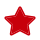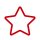# SELECT Subquery Syntax

#### The Subquery as Scalar Operand

```SELECT (SELECT id FROM test1 where id=1);
SELECT (SELECT id FROM test2 where id=1)FROM test1;
SELECT UPPER((SELECT name FROM test1 limit 1)) FROM test2;```

#### Comparisons Using Subqueries

```non_subquery_operand comparison_operator (subquery)
comparison_operator ：= > < >= <= <> != <=> like```

```select name from test1 where id > (select id from test2 where id=1);
select name from test1 where id = (select id from test2 where id=1);
select id from test1 where name like (select name from test2 where id=1);```

#### Subqueries with ANY, IN, NOT IN, SOME,ALL,Exists,NOT Exists

```operand comparison_operator SOME (subquery)
operand comparison_operator ALL (subquery)
operand comparison_operator ANY (subquery)
operand IN (subquery)
operand not IN (subquery)
operand exists (subquery)
operand not exists (subquery)```

```select id from test1 where id > any (select id from test2);
select id from test1 where id > some (select id from test2);
select id from test1 where id > all (select id from test2);
select id from test1 where id in (select id from test2);
select id from test1 where id not in (select id from test2);
select id from test1 where exists (select id from test2 where id=1);
select id from test1 where not exists (select id from test2 where id=1);```

#### Derived Tables (Subqueries in the FROM Clause)

`SELECT ... FROM (subquery) [AS] tbl_name ...`

`select id from (select id,name from test2 where id>1) a  order by a.id;`

#### 语法限制

• Derived Tables 必须拥有一个别名。
• Derived Tables 不可以成为 Correlated Subqueries，即不能包含子查询外部表的引用。
• 标量子查询在一些场景下当前不能得到正确结果，建议改写为join，同时可提高性能。
• 不支持 HAVING 子句中的子查询，JOIN ON 条件中的子查询。
• 不支持Row Subqueries。

# 相关产品